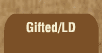Mathematics and Dyslexia

Tips for Learning the Times Tables

Tools for the Times Tables & Workbook

Home > Math > times tables practice > twos times tables in order

## The Twos Times Tables In Order

Numbers that you can divide by two are "even" because you can split that many things into two even piles. Even numbers alwaysend in 2, 4, 6, 8 or 0. If you multiply an even number by anything, the answer always even.

1. 2 x 0=
2. 2 x 1=
3. 2 x 2=
4. 2 x 3=
5. 2 x 4=
6. 2 x 5=
7. 2 x 6=
8. 2 x 7=
9. 2 x 8=
10. 2 x 9=
11. 2 x 10=
12. 6 x 2=
13. 2 x 7=
14. 8 x 2=
15. 2 x 9=